# Outline

As covered in the Get Started vignette, the function load_raster() loads raster data products in R as SpatRaster objects, which we can work with using the terra package. Let’s start by loading the seasonal relative abundance raster for the example Yellow-bellied Sapsucker data. We’ll work with the low resolution data in this vignette to ensure fast processing times.

library(ebirdst)
library(terra)
library(sf)
library(dplyr)
library(tidyr)
library(rnaturalearth)
library(geodata)
library(ggplot2)
library(fields)
extract <- terra::extract

# this simplified dataset doesn't require an access key
# alternatively, you can use the following to get the data path
path <- get_species_path("example_data")

# load seasonal mean relative abundance at low res
product = "abundance",
period = "seasonal",
metric = "mean",
resolution = "lr")

# get the seasons corresponding to each layer
names(abd_seasonal)
#>  "breeding"               "nonbreeding"            "prebreeding_migration"
#>  "postbreeding_migration"

# extract just the breeding season relative abundance
abd_breeding <- abd_seasonal[["breeding"]]

We can get the dates and quality scores associated with each of these seasons by filtering the ebirdst_runs data frame.

ebirdst_runs %>%
# note that the example data are for yellow-bellied sapsucker
filter(common_name == "Yellow-bellied Sapsucker") %>%
glimpse()
#> Rows: 1
#> Columns: 23
#> $species_code <chr> "yebsap" #>$ scientific_name                      <chr> "Sphyrapicus varius"
#> $common_name <chr> "Yellow-bellied Sapsucker" #>$ resident                             <lgl> FALSE
#> $breeding_quality <dbl> 3 #>$ breeding_range_modeled               <lgl> TRUE
#> $breeding_start <date> 2021-05-24 #>$ breeding_end                         <date> 2021-08-17
#> $nonbreeding_quality <dbl> 3 #>$ nonbreeding_range_modeled            <lgl> TRUE
#> $nonbreeding_start <date> 2021-11-23 #>$ nonbreeding_end                      <date> 2021-03-08
#> $postbreeding_migration_quality <dbl> 3 #>$ postbreeding_migration_range_modeled <lgl> TRUE
#> $postbreeding_migration_start <date> 2021-08-24 #>$ postbreeding_migration_end           <date> 2021-11-16
#> $prebreeding_migration_quality <dbl> 3 #>$ prebreeding_migration_range_modeled  <lgl> TRUE
#> $prebreeding_migration_start <date> 2021-03-15 #>$ prebreeding_migration_end            <date> 2021-05-17
#> $resident_quality <dbl> 3 #>$ resident_start                       <date> NA
#> $resident_end <date> NA # Mapping relative abundance In this section, we’ll demonstrate how to make a simple map of breeding season relative abundance. However, note that to make high-quality, publication-ready maps typically requires extra work. It many cases, it may be worthwhile designing maps in a traditional GIS environment such as QGIS or ArcGIS. The simplest way to map the seasonal relative abundance data is to use the built in plot() function from the raster package. plot(abd_breeding, axes = FALSE)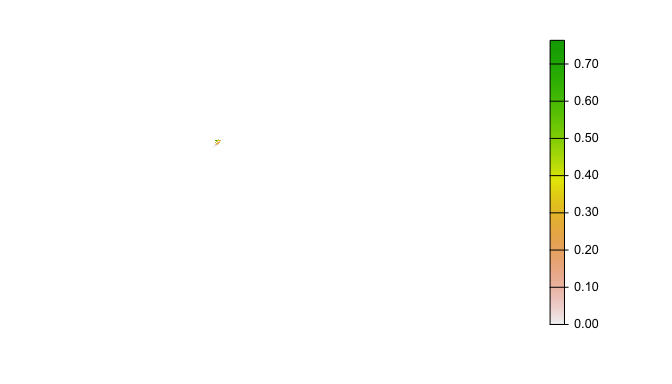Clearly this approach doesn’t work out of the box! There are a wide variety of issues that we’ll tackle one at a time. ## Extent All raster data downloaded through this package are defined over the same global grid, regardless of the range of the individual species. The example data only has non-zero abundance within the state of Michigan, which is barely visible on the above global map. We need to define an extent for our map. There are a variety of ways to do this, but we’ll use the R package rnaturalearth to access a polygon boundary for Michigan, which we’ll then use to crop the raster. # boundary polygon for michigan mi <- ne_states(iso_a2 = "US", returnclass = "sf") %>% filter(postal == "MI") %>% # project to same coordinate reference system as the raster data st_transform(st_crs(abd_seasonal)) # crop data to michigan abd_breeding_mi <- crop(abd_breeding, mi) # map the cropped data plot(abd_breeding_mi, axes = FALSE)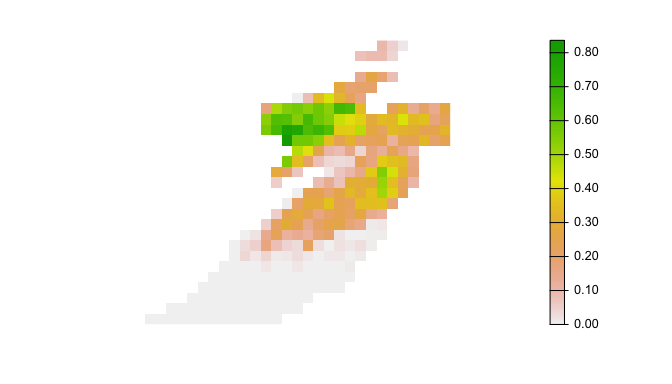## Projection The raster data are all provided in the same equal area sinusoidal projection as NASA MODIS data. While this projection is suitable for analysis, it is not ideal for mapping since it introduces significant distortion. Instead, as part of the Status and Trends workflow, custom species-specific projections are provided that are optimized for the region that the species occurs within. We can access the projection for Yellow-bellied Sapsucker with load_fac_map_parameters(), then transform the raster data to this custom projection. # load the mapping parameters fac_parameters <- load_fac_map_parameters(path) crs <- fac_parameters$custom_projection

# transform to the custom projection using nearest neighbor resampling
abd_projected <- project(abd_breeding_mi, crs, method = "near")

# map the cropped and projected data
plot(abd_projected, axes = FALSE)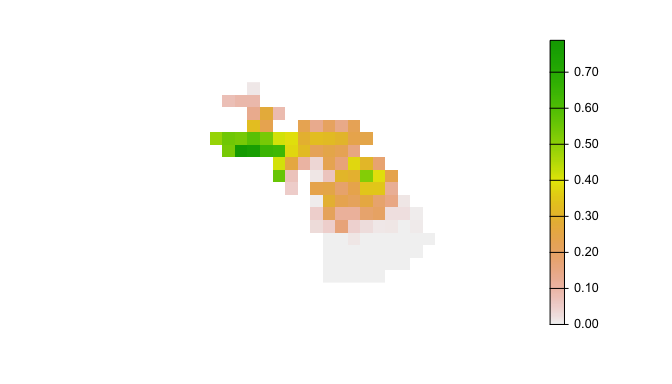## Abundance bins

The relative abundance data are not uniformly distributed, which can lead to challenges distinguishing areas of differing levels of abundance. To address this, we’ll use a quantile bins for the map, where each color in the legend corresponds to an equal number of cells in the raster. We’ll define these bins excluding zeros, then assign a separate color to the zeros. We can also use the function abundance_palette() to get the same set of colors we use in the legends on the eBird Status and Trends website.

# quantiles of non-zero values
v <- values(abd_projected)
v <- v[!is.na(v) & v > 0]
bins <- quantile(v, seq(0, 1, by = 0.1))
# add a bin for 0
bins <- c(0, bins)

# status and trends palette
pal <- abundance_palette(length(bins) - 2)
# add a color for zero
pal <- c("#e6e6e6", pal)

# map using the quantile bins
plot(abd_projected, breaks = bins, col = pal, axes = FALSE)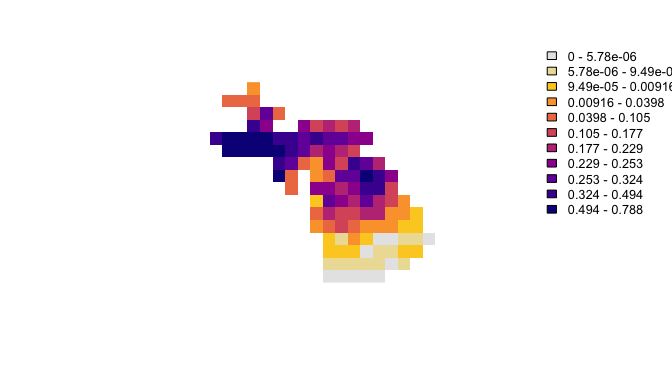## Basemap

Finally, we’ll add state and country boundaries to provide some context. The R package rnaturalearth is an excellent source of attribution free contextual GIS data.

# natural earth boundaries
countries <- ne_countries(returnclass = "sf") %>%
st_geometry() %>%
st_transform(crs)
states <- ne_states(iso_a2 = "US", returnclass = "sf") %>%
st_geometry() %>%
st_transform(crs)

# define the map extent with the michigan polygon
mi_ext <- mi %>%
st_geometry() %>%
st_transform(crs)
plot(mi_ext)
plot(countries, col = "#cfcfcf", border = "#888888", add = TRUE)
plot(abd_projected,
breaks = bins, col = pal,
axes = FALSE, legend = FALSE, add = TRUE)
plot(countries, col = NA, border = "#888888", lwd = 3, add = TRUE)
plot(states, col = NA, border = "#888888", add = TRUE)

# add legend using the fields package
# label the bottom, middle, and top
labels <- quantile(bins, c(0, 0.5, 1))
label_breaks <- seq(0, 1, length.out = length(bins))
image.plot(zlim = c(0, 1), breaks = label_breaks, col = pal,
smallplot = c(0.90, 0.93, 0.15, 0.85),
legend.only = TRUE,
axis.args = list(at = c(0, 0.5, 1),
labels = round(labels, 2),
col.axis = "black", fg = NA,
cex.axis = 0.9, lwd.ticks = 0,
line = -0.5))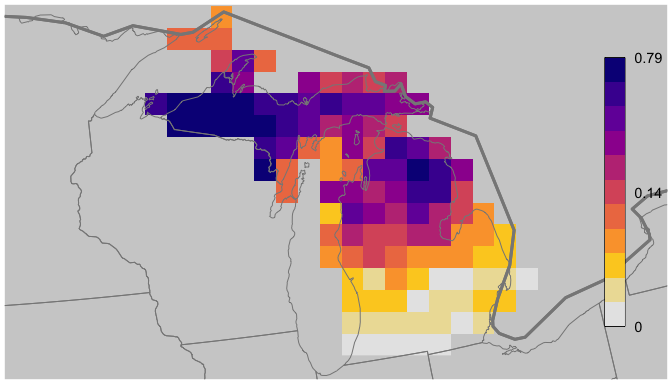# Extracting trajectories with uncertainty

Next, we’ll look at the temporal component of the relative abundance data. Using the weekly relative abundance cubes, we can chart the change in relative abundance throughout the year for a fixed location. Furthermore, using the upper and lower confidence interval rasters, we can add uncertainty estimates. We often refer to these as relative abundance trajectories.

abd_median <- load_raster(path, product = "abundance",
metric = "median", resolution = "lr")
abd_lower <- load_raster(path, product = "abundance",
metric = "lower", resolution = "lr")
abd_upper <- load_raster(path, product = "abundance",
metric = "upper", resolution = "lr")

Now we’ll extract the values for a fixed location.

# set a point
pt <- st_point(c(-88.1, 46.7)) %>%
st_sfc(crs = 4326) %>%
st_transform(crs = st_crs(abd_median)) %>%
st_coordinates()

# extract
traj_median <- as.matrix(extract(abd_median, pt))[1, ]
traj_upper <- as.matrix(extract(abd_upper, pt))[1, ]
traj_lower <- as.matrix(extract(abd_lower, pt))[1, ]

# plot trajectories
plot_frame <- data.frame(x = seq_len(length(traj_median)),
y = unname(traj_median),
lower = unname(traj_lower),
upper = unname(traj_upper))
ggplot(plot_frame, aes(x, y)) +
geom_line(data = plot_frame) +
geom_ribbon(data = plot_frame,
aes(ymin = lower, ymax = upper),
alpha = 0.3) +
ylab("Relative abundance") +
xlab("Week") +
theme_light()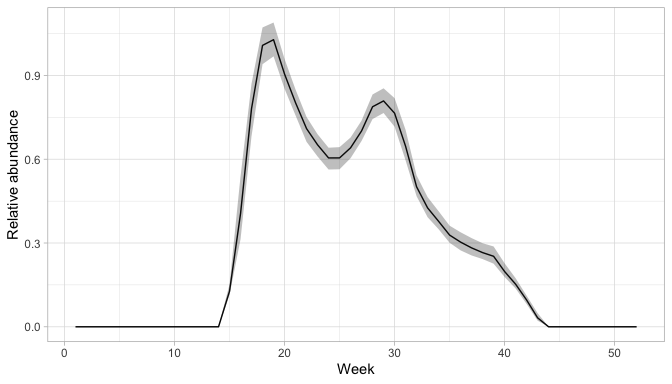# Regional statistics

In addition to maps and visualizations, the eBird Status and Trends website provides a set of statistics summarizing the spatial data over regions (countries and states) and seasons. The five regional statistics are:

1. Mean relative abundance: the average estimated relative abundance within the given region.
2. Percentage of seasonal population: the sum of the estimated relative abundance within the selected region divided by the sum of the estimated relative abundance across the full range.
3. Percentage of region occupied: the percentage of the selected region within the range boundary of a species.
4. Percentage of range in region: the fraction of a species’ total range that falls within the selected region.
5. Days of occupation in region: the number of days that a species occupies the selected region, with occupation being defined as spatially covering the selected region by at least 5% based on estimated relative abundances averaged across the given season.

These statistics can be downloaded from the Status and Trends website for all regions and seasons for any given species; however, there may be situations where you want to calculate them over different regions than those provided. With that in mind, in this section we’ll cover how to calculate a couple of these statistics: percent of population in region and percent of region occupied. The remaining 3 statistics can be calculated following the same approach with some modifications.

Since the example data used in this vignette is restricted to Michigan, we’ll calculate the statistics over the counties in Michigan; however, this approach can easily be extended to any set of regions. Let’s start by downloading county boundaries for Michigan.

mi_counties <- gadm(country = "USA", level = 2, path = tempdir()) %>%
st_as_sf() %>%
filter(NAME_1 == "Michigan") %>%
select(county = NAME_2, county_code = HASC_2) %>%
# remove lakes which aren't true counties
filter(county_code != "US.MI.WB")
# project to sinusoidal
mi_counties_proj <- st_transform(mi_counties, crs = st_crs(abd_median))

We’ll need the seasonal percent of population cubes and the seasonal ranges for these calculations.

pop_seasonal <- load_raster(path, product = "percent-population",
period = "seasonal", resolution = "lr")
ranges <- load_ranges(path, resolution = "lr", smoothed = FALSE)

## Percent of Population

Percent of population in regions is one of the simplest statistics to calculate since a raster of percent of population is already provided; we simply sum all the raster cells within each region polygon.

pctpop <- extract(pop_seasonal, mi_counties_proj, fun = sum, na.rm = TRUE) %>%
# attach county attributes
mutate(county_code = mi_counties$county_code) %>% # transpose to long format, one season per row select(-ID) %>% pivot_longer(cols = -county_code, names_to = "season", values_to = "percent_population") head(pctpop) #> # A tibble: 6 × 3 #> county_code season percent_population #> <chr> <chr> <dbl> #> 1 US.MI.AC breeding 0.00156 #> 2 US.MI.AC nonbreeding 0 #> 3 US.MI.AC prebreeding_migration 0.00105 #> 4 US.MI.AC postbreeding_migration 0.00134 #> 5 US.MI.AG breeding 0.00178 #> 6 US.MI.AG nonbreeding 0 Let’s make a quick map comparing the breeding and non-breeding percent of population within counties in Michigan. # join back to county boundaries pctpop_proj <- pctpop %>% filter(season %in% c("breeding", "nonbreeding")) %>% inner_join(mi_counties, ., by = "county_code") %>% # transform to custom projection for plotting st_transform(crs = crs) # plot ggplot(pctpop_proj) + geom_sf(aes(fill = percent_population)) + scale_fill_viridis_c(trans = "sqrt") + guides(fill = guide_colorbar(title.position = "top", barwidth = 15)) + facet_wrap(~ season, ncol = 2) + labs(title = "Seasonal percent of population in MI counties", fill = "Percent of population") + theme_bw() + theme(legend.position = "bottom")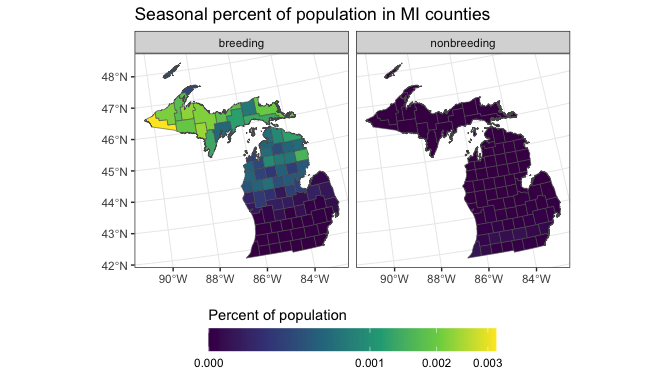## Percent of region occupied To calculate range-based stats it’s often easiest to use the range polygons rather than the raster data. We can calculate the area of each county, then calculate the area of intersection between the counties and the ranges, and finally divide the two to get the percent of each region occupied. # add the area of each region mi_counties$area <- st_area(mi_counties)
# for each season, intersect with the county boundaries and calculate area
range_pct_occupied <- NULL
for (s in ranges\$season) {
range_pct_occupied <- ranges %>%
filter(season == s) %>%
st_intersection(mi_counties, .) %>%
mutate(percent_occupied = as.numeric(st_area(.) / area)) %>%
select(season, county_code, percent_occupied) %>%
st_drop_geometry() %>%
bind_rows(range_pct_occupied, .)

}
#> 6 breeding    US.MI.AR        1.0000000